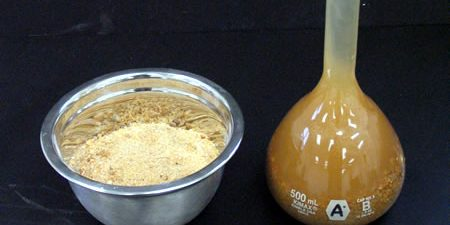# Specific Gravity and Absorption of Fine Aggregate Determination

Introduction:

The specific gravity of fine aggregate is an important parameter that can affect the performance of concrete mixtures. The pycnometer method is one of the most commonly used methods for determining the specific gravity of fine aggregate. This method involves filling a pycnometer with water, introducing a sample of fine aggregate into the pycnometer, and measuring the mass of the pycnometer and its contents. The specific gravity of the fine aggregate is then calculated based on these measurements. This procedure is based on the ASTM C128 standard test method.

## Objectives:

• To determine the specific gravity of fine aggregate using the pycnometer method
• To ensure accurate and precise measurements are taken throughout the procedure

## Equipment Needed:

1. Pycnometer
2. Water
3. Oven
4. Weighing scale
5. Fine aggregate sample

## Procedure:

1. Partially fill the pycnometer with water and record the mass.
2. Introduce 500 ± 10 g of saturated surface-dry fine aggregate into the pycnometer and fill with additional water to approximately 90% of capacity. (Learn the Procedure for Attaining Saturated Surface Dry Conditions of Fine Aggregate)
3. Agitate the pycnometer manually or mechanically to eliminate any visible air bubbles (refer to Section 9.2.1.1 and 9.2.1.2 of ASTM C128).
4. Adjust the temperature of the pycnometer and its contents to 23.0 ± 2.0 °C if necessary by partial immersion in circulating water, and bring the water level in the pycnometer to its calibrated capacity.
5. Determine the total mass of the pycnometer, specimen, and water.
6. Remove the fine aggregate from the pycnometer and dry it in an oven to constant mass at a temperature of 110 ± 5 °C.
7. Cool the dried fine aggregate in air at room temperature for 1 ± 1⁄2 hours and determine the mass.
8. Determine the mass of the pycnometer filled to its calibrated capacity with water at 23.0 ± 2.0 °C.
9. Calculate the specific gravity of the fine aggregate using the following formula:

## Results and Calculations

Record all measurements and calculations in a laboratory notebook. Report the specific gravity and absoprtion of the fine aggregate to the nearest 0.01.

• A = mass of oven dry specimen
• B = mass of pycnometer filled with water, to calibration mark
• C = mass of pycnometer filled with specimen and water to calibration mark
• S = mass of saturated surface-dry specimen

Oven dry specific gravity = A / (B + S – C)

SSD specific gravity = S / (B + S – C)

Apparent specific gravity = A / (B + A – C)

Absorption = 100 * [(S – A)/A]

## Discussion and Conclusion:

The specific gravity of fine aggregate is an important property that helps in determining the weight-to-volume relationship of concrete. A higher specific gravity indicates that the aggregate is denser and can lead to a heavier mix, while a lower specific gravity can lead to a lighter mix. The range of specific gravity for fine aggregate is typically between 2.4 to 2.9.